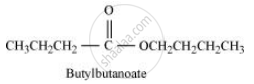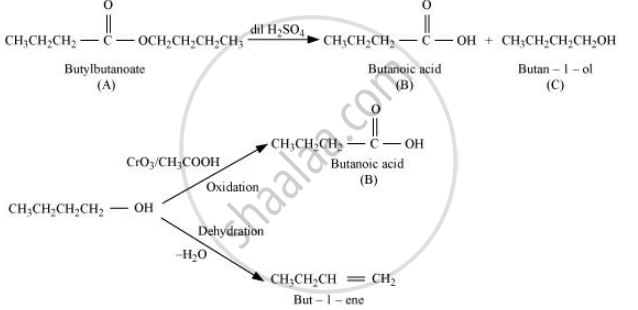Department of Pre-University Education, KarnatakaPUC Karnataka Science Class 12
Share

# An organic compound (A) (molecular formula C8H16O2) was hydrolysed with dilute sulphuric acid to give a carboxylic acid (B) and an alcohol (C). Oxidation of (C) with chromic acid produced (B). (C) on dehydration gives but-1-ene.Write equations for the reactions involved. - Chemistry

#### Question

An organic compound (A) (molecular formula C8H16O2) was hydrolysed with dilute sulphuric acid to give a carboxylic acid (B) and an alcohol (C). Oxidation of (C) with chromic acid produced (B). (C) on dehydration gives but-1-ene.Write equations for the reactions involved.

#### Solution

An organic compound A with molecular formula C8H16O2 gives a carboxylic acid (B) and an alcohol (C) on hydrolysis with dilute sulphuric acid. Thus, compound A must be an ester. Further, alcohol C gives acid B on oxidation with chromic acid. Thus, B and C must contain equal number of carbon atoms.

Since compound A contains a total of 8 carbon atoms, each of B and C contain 4 carbon atoms.

Again, on dehydration, alcohol C gives but-1-ene. Therefore, C is of straight chain and hence, it is butan-1-ol.

On oxidation, Butan-1-ol gives butanoic acid. Hence, acid B is butanoic acid.

Hence, the ester with molecular formula C8H16O2 is butylbutanoate.All the given reactions can be explained by the following equations.Is there an error in this question or solution?